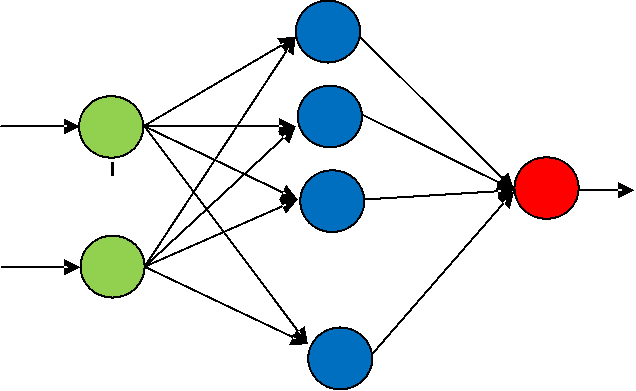Nghiên cứu khoa học công nghệ
SECOND ORDER NONLINEAR SYSTEM
BASED ON RBF NEURAL NETWORK
Nguyen Dang Tien
Abstract: In this paper, a novel control method for second order nonlinear
system based on sliding mode controller, RBF neural network and adaptive control
system is proposed. Firstly, a new controller is designed based on sliding mode
control system. Then, a RBF neural network and an adaptive system are integrated
to estimate unknown parameters of the system. Moreover, in order to deal with
chattering phenomenon in conventional sliding controller, boundary layer method
is then applied. Finally, the experimental results are presented to demonstrate the
effectiveness of the proposed control method.
Keywords: RBF neural network, Sliding mode controller, Adaptive controller, Chattering phenomenon,
Boundary layer method.
1. PROBLEM STATEMENT
The nonlinear controller which uses linear regression method has been used
extensively by researchers when the mathematic model of the object is known .
There
are
many
systems
which
have
been
successfully
applied
the
controller to solve the problem of noise [2-4]. However, adaptive controller is not
the optimal solution for nonlinear system which is heavily affected by chattering
phenomenon of noisy environment [5-7].
Neural network is one of the best solution for complicated systems which
affected
by
noise
due
to
the
capability
of
learning
and
approximating
any
nonlinear formulas. RBF neural is a special case of neural network with superior
characteristics compare to traditional neural network such as: simple structure,
fast learning algorithm and good approximation. Moreover, RBF neural network
is not only able of eliminating local minimum, reduce the number of parameters
in
the
network
but
also
make
the
initialization
process
become
significantly
easier. Therefore, this network has been successfully applied in many types of
controllers [8-10].
Recently, sliding mode controller is known to be the best methods for solving
the problem of noise affected by surrounding environment. The advantages of
sliding mode control such as: stability in noisy environment, ability to respond
quickly and good quality of control. However, in this method, when the effect of
noise is high, we have to apply a large gain in discrete control signal in order to
make the system operating normally. This creates the chatting phenomenon which
can cause the damage for electronic equipment and make the system becomes
unstable. In this paper, to overcome all the above issues, we propose a new control
method based on the integration of three components: adaptive controller, sliding
mode controller and RBF neural network.
Firstly, the controller is designed based
on the model of sliding controller. Then, to reduce the effect of noise, an adaptive
compensator which uses RBF neural network is proposed. Finally, boundary layer
method is applied to solve the chattering phenomenon.
Tạp chí Nghiên cứu KH&CN quân sự, Số 48, 04 - 2017
79x
w
w
T
1
2b
2
i
L
i
 
1
2
1
Kỹ thuật điều khiển & Điện tử
2. PROBLEM ESTABLISHMENT
2.1. RBF neural network
A normal RBF neural network is constructed by three layers as follow (Fig. 1).
Hidden layer
Input layer
1
Output layer
1
1
1
w2
3
xN
wL
N
L
Figure 1. RBF neural network.
Input layer: Is also the input vector and can be described as follow
x=[x ,x2,...,xN ]
(1)
Hidden layer: Is the second layer and has the conversion function as follow
i(x)=exp((xci)2 ),
i
i =1,2,...,L
(2)
where
x
is the input vector, ci
is the eccentricity of Gaussian distribution, b
is
variance of Gaussian distribution and L is the number of neural in hidden layer.
Output layer: Output layer of RBF neural network can be calculated by sum of
all weighted input signals.
y= wi(x)+
i=0
(3)
2.2. Second order nonlinear system
The dynamic equation of second order nonlinear system is described as follow
x= f (x,x)+mu
(4)
where
f (x,x) is an unknown nonlinear function, m is an unknown real number
and u is the control signal.
The dynamic equation (4) can be rewritten as follow
x = x2
x = f (x)+mu
y = x
(5)
80
N. Đ. Tiến, “Adaptive sliding mode controller for… based on RBF neural network.1
T
1
T
1
1

ˆ
ˆ
ˆ
ˆ
ˆ
1
ˆ
ˆ
ˆ
Nghiên cứu khoa học công nghệ
where x =[x ,x2 ]T .
The aim of this paper is: designing a stable controller to make sure that the real
value of nonlinear system y will be closed to the designed value
yd
in such a
condition that we don’t know the value of
f (x) and m.
2.3. Controller design
Set e(t)= yd (t)y(t) is the error vector of the controller. To establish the sliding
controller, firstly, we have to design the sliding surface.
s=e+ 1e
(6)
where
1 =diag( 11, 12,..., 1n),
with1i >0
,
i =1,2,...n
and
s=[s ,s2,...,sn] ,
e=[e,e2,...,en] . It is clearly to see that
s=0
is a stable sliding surface
e0,
e0 as t .
According to the process of designing sliding mode controller, the control signal
contains below components
=ueq +uSW =ueq +KSWsign(s)
(7)
where KSW =diag(kSW1,kSW 2,...,kSWn) and kSW1,kSW2,...kSWn are positive values. The
equivalent control signal ueq can be calculated when s=0.
ueq = m(f (x)+yd +KT E)
(8)
where K =kp
kd T and kp,kd are positive values, E=e
e .
It is obvious to see that the control signal contains the discrete signal (which
causes
the
chattering
phenomenon).
Therefore,
to
overcome
this
problem,
we
propose a new controller which uses a RBF neural network to approximate f (x) ,
an adaptive controller to estimate the value of m. The discrete signal is then
processed by boundary layer method.
f(x)=WTh(x)
(9)
where
f (x)
is
the
approximate
function
of
f (x),
WT
is
weighted
estimator, h(x) is the output of hidden layer.
Adaptive parameters are calculated as follow
W =−ET PBh(x)
(10)
ET PBu
m=1 ET PBu
1
if ET PBu>0
if ET PBu0 &m>m
if ET PBu0 &m<m
(11)
Tạp chí Nghiên cứu KH&CN quân sự, Số 48, 04 - 2017
81